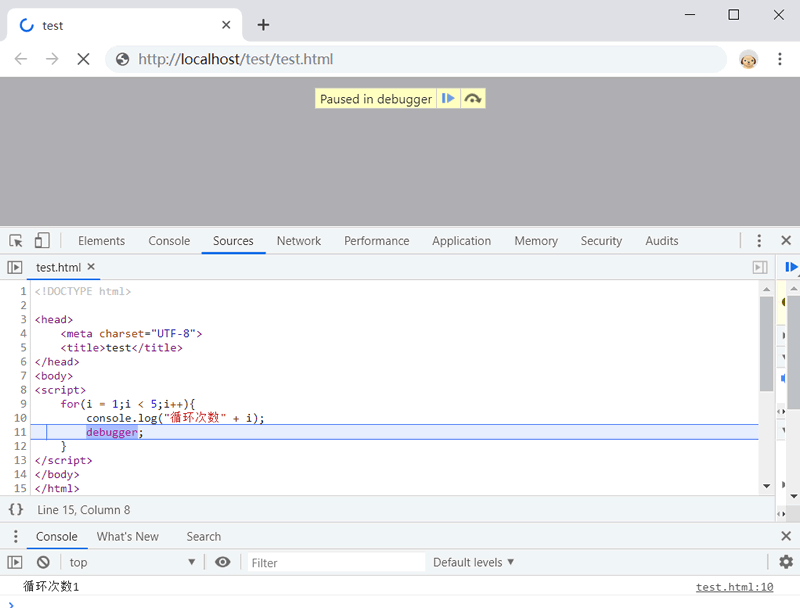## JS语句完全攻略

• 内容
• 评论
• 相关

JavaScript 语言定义了 20 套（或个）语句命令，分别执行不同的操作。

• 单句：也称单行语句，由一个或多个关键字和表达式构成，用来完成简单的运算。
• 复句：使用大括号包含一个或多个单句，用来设计代码块、控制流程等复杂操作。

## 定义语句

`var a;`

```var a
a = 1```

`var a a = 1`

JavaScript 无法理解这句话的意思，于是添加分号，定义为两条语句来解析。但是，对于下面 3 行代码：

```var b = ""
var a = b
(a = "abc").toUpperCase()```

```var b = "";
var a = b(a = "abc").toUpperCase();```

#### 提示：

```a
.length  //上下行合并解析为：a.length
a
/b/  //上下行合并解析为：a/b/
a
-1  //上下行合并解析为：a-1
a
+1  //上下行合并解析为：a+1```

1) return、break 和 continue 三个语句，如果分行显示，JavaScript 不会自动合并下一行进行解析。例如：

```return
1;  //不会合并，直接解析为两条语句：return 和 1；```

2) ++(递增)和 --（递减）运算符会与下一行变量主动合并解析，但不会与上一行变量合并解析。例如：

```var a = b = 1;
a  //结果为1
++
b  //结果为2```

• 空语句
• 表达式语句
• 声明语句
• 调试语句
• 启用严格模式语句

## 复句

#### 示例

```{
//空复句
}
{
console.log("单复句);
}
{
console.log("外层复句");
{
console.log("内层复句");
}
}```

## 空语句

`for(var i = 0;i < 10;i++){ }`

`for(var i = 0;i < 10;i++);`

```for(var i = 0;i < 10;i++)/*空语句*/；
for(var i = 0;i < 10;i++){ ; }```

## 表达式语句

#### 示例1

`true;  //最简单的句子`

#### 示例2

```o = new ((o == "String") ? String : (o == "Array") ? Array : (o ==
"Number") ? Number : (o == "Math") ? Math : (o == "Date") ? Date : (o ==
"Boolean") ? Boolean : (o == "RegExp") ? RegExp : Object);```

```new ((o == "String") ? String  :
(o == "Array") ? Array  :
(o == "Number") ? Number  :
(o == "Math") ? Math  :
(o == "Date") ? Date  :
(o == "Boolean") ? Boolean  :
(o == "RegExp") ? RegExp  :
Object);```

• 子句法角度分析，表达式是短句；语句是一个句子。
• 从结构角度分析，表达式由操作数和运算符组成；语句由命令（关键字）和表达式组成。表达式之间可以通过空格分隔；而语句之间必须通过分号分隔。表达式可以包含子表达式，语句也可以包含子语句。
• 从表现角度分析，表达式呈现静态性；而语句呈现动态性。
• 从结果趋向分析，表达式必须返回一个值；而语句则是完成特定操作。

## 声明语句

#### 示例1

`var a = 0,b = true,c,d;  //声明四个变量，并部分赋值`

#### 示例2

```function f() {
console.log("声明函数");
}```

## 调试语句

debugger 语句用于停止执行 JavaScript，同时如果调试函数可用，会调用调试函数。

debugger 语句可以放在代码的任何位置用以中止脚本执行，但不会关闭任何文件或清除任何变量，类似于在代码中设置断点。

```for(i = 1;i < 5;i++){
console.log("循环次数" + i);
debugger;
}```0条评论